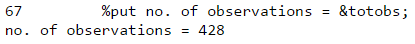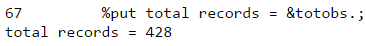# Check number of observations in SAS dataset

This post explains how to determine the number of observations in a SAS dataset. Most of the times we need to check whether a SAS dataset is empty or not. In macro, we generally tell SAS to go to the next iteration only when SAS dataset is non-empty. In this post, we will see various methods to count number of  rows (records) in SAS table.

Method I : Proc SQL Count (Not Efficient)

In the example below, we will use CARS dataset from SASHELP library. This dataset contains 428 observations and 15 columns.

The easiest method is to use count(*) in Proc SQL. It returns all rows (missing plus non-missing rows) in a dataset.
proc sql;
select count(*) as N from sashelp.cars;
quit;
Result : 428

In case you want to store it in a macro variable, you can use INTO : keyword.
proc sql noprint;
select count(*) into :N from sashelp.cars;
quit;

%put &N;
This will print the number of records in SAS log. Check log after running the above program.

Is it an efficient method?
No, it is not efficient at all. It does not use metadata information of SAS dataset. Instead it reads through each record (row) of your SAS dataset. It takes a long time to do it in big SAS tables. However, it is a simple and handy trick to calculate the number of rows in a SAS dataset.

Method 2 : Descriptor Portion (Efficient)

Before getting into detail, we need to understand the descriptor portion and how it works -

SAS dataset consists of the following two portion -
1. Descriptor portion. It constitutes information about name of dataset, number of observations and variables, creation date, engine type.
2. Data portion. It stores values of data.
This method is one of the most efficient way to count observations in a SAS table as it uses metadata information and does not search in dataset.
data _NULL_;
if 0 then set sashelp.cars nobs=n;
put "no. of observations =" n;
stop;
run;
Explanation
1. The 'if 0' statement  does not process at execution time because IF statement does not hold TRUE. The whole IF THEN statement is used to pull the header information of the data set and later hand over to the compiler to adjust it to the PDV.
2. NOBS is a SAS automatic variable which contains the number of rows in a dataset i.e. SASHELP.CARS dataset.
3. NOBS = N puts the returns count of records in the variable n.
4. The STOP statement is used to stop an endless loop.
Like the first method, we can keep it in a macro variable. See the implementation below -
data _NULL_;
if 0 then set sashelp.cars nobs=n;
call symputx('totobs',n);
stop;
run;
%put no. of observations = &totobs;SAS Output

CALL SYMPUT is one of the method to create a SAS macro variable in data step. In this case, we have used a newer function i.e. CALL SYMPUTX which left justifies and trims trailing blanks from a numeric value. If you want to stick to the old style CALL SYMPUT, you can write like below -
call symput('totobs',left(n));

3. Proc SQL Dictionary Method (Efficient)

Like second method, we can use metadata information of a dataset with PROC SQL Dictionary.Tables.
proc sql noprint;
select nobs into :totobs separated by ' ' from dictionary.tables
where libname='SASHELP' and memname='CARS';
quit;
%put total records = &totobs.;Proc SQL Dictionary.Tables

It is an efficient method as it does not look into each values of  a dataset to determine the count. The LIBNAME= refers to the name of the library in which data is stored. The MEMNAME= refers to SAS table (dataset). The separated by ' ' is used in this case to left align the numeric value.

4. Macro Language Method (Efficient)

This method also uses metadata information but it is via the macro language using DATA step functions. The OPEN function is used to open a data. The ATTRN function returns the value of a numeric attribute for a SAS data set. When it is used with the NOBS argument, it returns the number of observations. Later we are closing the opened dataset using CLOSE function.
%macro totobs(mydata);
%let mydataID=%sysfunc(OPEN(&mydata.,IN));
%let NOBS=%sysfunc(ATTRN(&mydataID,NOBS));
%let RC=%sysfunc(CLOSE(&mydataID));
&NOBS
%mend;
%put %totobs(sashelp.cars);

SAS : Check if it is empty table

Suppose you only need to check whether a table is empty or not. You can use the same logic as explained above. And if the returned value is 0, write 'Empty Data' in log. Otherwise, count the number of records.
data _NULL_;
if 0 then set sashelp.cars nobs=n;
if n = 0 then put 'empty dataset';
else put 'Not empty. Total records=' n;
stop;
run;
Result : Not Empty. Total records = 428

Let's create a blank dataset to check the above code. The following program returns empty dataset as 1=2 condition does not meet.
proc sql noprint;
create table temp as
select * from sashelp.cars
where 1 = 2;
quit;
Try it yourself!

Let's wrap the above code in a SAS macro
%macro emptydataset (inputdata=);
data _NULL_;
if 0 then set &inputdata. nobs=n;
call symputx('totobs',n);
stop;
run;
%if &totobs. = 0 %then %put Empty dataset;
%else %do;
%put TotalObs=&totobs;
%end;
%mend;
%emptydataset(inputdata=sashelp.cars);
Result : TotalObs=428

%emptydataset(inputdata=work.temp);
Result : Empty dataset

If you think it's difficult to memorize sas code of descriptor portion method, you can use the code below.
data _NULL_;
set sashelp.cars nobs=N;
if _N_ = 2 then stop;
put N;
run;SAS log
It reads only first two observations from the dataset. See log above.Share
Related PostsDeepanshu founded ListenData with a simple objective - Make analytics easy to understand and follow. He has over 10 years of experience in data science. During his tenure, he has worked with global clients in various domains like Banking, Insurance, Private Equity, Telecom and Human Resource.

8 Responses to "Check number of observations in SAS dataset"
1.Hi,
Can you please help me in deleting all the empty data sets of any library without opening it or how to check if particular data set is empty or not.

Thanks

1.Create macro var from proc SQL and use dictionary.tables where nobs=0 then use proc datasets and after delete values use ref to that macro var

2.give me your email, let me send you the code

2.Hi Deepanshu, very useful post

I have a question about "model scoring" task. I have created and registered a model in Enterprise Miner, now I am scoring
this model from Enterprise Guide using "model scoring" task.
In the output variables of the model I have three types of var:
P_targetvar Q_targetvar U_targetvar V_targetvar

I think that P_targetvar is the predicted value for target variable of the model, but I would like to know the
meaning of Q_, U_ and V_ vars.

Another question I would like to know if I can definde and use a Enterprise Miner model with parameters. I mean not only
the vars of the dataset, this parameters would be about the type of model (selection criteria, type of decision tree...)

Juan

3.Thnaks good presentation

4.Excellent site for knowing SAS knowledge. :)

5.Very Informative

6.Good information, thanks

Next → ← Prev